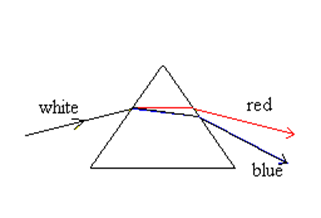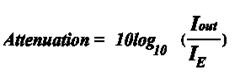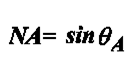# Fiber Optic Theory (3)

The data which is carried in an optical fiber consists of pulses of light energy following each other rapidly. There is a limit to the highest frequency, i.e. how many pulses per second which can be sent into a fiber and be expected to emerge intact at the other end. This is because of a phenomenon known as pulse spreading which limits the “Bandwidth” of  the fiber.The pulse sets off down the fiber with an nice square wave shape. As it travels along the fiber it gradually gets wider and the peak intensity decreases.

The cause of cause spreading is dispersion. This means that some components of the pulse of light travel at different rates along the fiber. there are two forms of dispersion.
1. Chromatic dispersion
2. Modal dispersion

Chromatic Dispersion

Chromatic dispersion is the variation of refractive index with the wavelength (or the frequency) of the light. Another way of saying this is that each wavelength of light travels through the same material at its own particular speed which is different from that of other wavelengths.

For example, when white light passes through a prism some wavelengths of light bend more because their refractive index is higher, i.e. they travel slower This is what gives us the “Spectrum” of white light. The “red’ and “orange” light travel  slowest and so are bent most while the “violet” and “blue” travel fastest and so are bent less. All the other colors lie in between.

This means that different wavelengths travelling through an optical fiber also travel at different speeds. This phenomenonis called “Chromatic Dispersion”.Dispersion of Light through a Prism

Modal Dispersion

In an optical fiber there is another type of dispersion called “Multimode Dispersion”.

More oblique rays (lower order modes) travel a shorter distance. These correspond to rays travelling almost parallel to the center line of the fiber and reach the end of fiber sooner. The more zig-zag rays (higher order modes) take a longer  route as they pass along the fiber and so reach the end of the fiber later.

Now:Total dispersion = chromatic dispersion + multimode dispersion

Or put simply: for various reasons some components of a pulse of light travelling along an optical fibre move faster and other components move slower. So, a pulse which starts off as a narrow burst of light gets wider because some components race ahead while other components lag behind, rather like the runners in a marathon race.

Frequency Limit (Bandwidth)

The further the pulse travels in the fiber the worse the spreading gets,Pulse spreading limits the maximum frequency of signal which can be sent along a fiber. If signal pulses follow each other too fast then by the time they reach the end fiber they will have merged together and become indistinguishable. This is unacceptable for digital systems which depend on the precise sequence of pulses as a code for information. The Bandwidth is the highest number of pulses per second, that can be carried by the fiber without loss of information due to pulse spreading.

Distance Limit

A given length of fiber,as explained above has a maximum frequency (bandwidth) which can be sent along it. If we want to increase the bandwidth for the same type of fiber we can achieve this by decreasing the length of the fiber. Another way of saying this is that for a given data rate there is a maximum distance which the data can be sent.

Bandwidth Distance Product (BDP)

We can combine the two ideas above into a single term called the bandwidth distance product (BDP). It is the bandwidth of a fiber multiplied by the length of the fiber. The BDP is the bandwidth of a kilometer of fiber and is a constant for any particular type of fiber. For example, suppose a particular type of multimode fiber has a BDP of 20 MHz.km, then:

• 1 km of the fiber would have a bandwidth of 20MHz
• 2 km of the fiber would have a bandwidth of 10MHz
• 5 km of the fiber would have a bandwidth of 4MHz
• 4 km of the fiber would have a bandwidth of 5MHz
• 10 km of the fiber would have a bandwidth of 2MHz
• 20 km of the fiber would have a bandwidth of 1MHz

The typical B.D.P. of the three types of fibers are as follows:

Multimode 6 – 25 MHz.km

Single Mode 500 – 1500 MHz.km

Graded Index 100 – 1000 MHZ.km

NB: The units of BDP are MHz.km (read as megahertz kilometers). They are not MHz/km (read as megahertz per kilometers). This is because the quantity is a product (of bandwidth and distance) and not a ratio.

Choice of fiber

Multimode fiber

Muitimode fiber is suitable for local area networks (LAN’s) because it can carry enough energy to support all the  subscribers to the network. In a LAN the distances involved, however, are small. Little pulse spreading can take place and so the effects of dispersion are unimportant.

Single Mode fiber

Multimode Dispersion is eliminated by using Single Mode fiber. The core is so narrow that only one mode can travel. So the amount of pulse spreading in a single mode fiber is greatly reduced from that of a multimode fiber. Chromatic dispersion however remains even in a single mode fiber. Thus even in single mode fiber pulse spreading can occur. But chromatic dispersion can be reduced by careful design of the chemical composition of the glass.

The energy carried by a single mode fiber, however, is much less than that carried by a multimode fiber. For this reason single mode fiber is made from extremely low loss, very pure, glass.

Single mode low absorption fiber is ideal for telecommunications because pulse spreading is small.

In graded index fiber rays of light follow sinusoidal paths. This means that low order modes, i.e. oblique rays, stay close  to the center of the fiber, high order modes spend more time near the edge of core. Low order modes travel in the high  index part of the core and so travel slowly, whereas high order modes spend.

Predominantly more time in the low index part of the core and so travel faster. This way, although the paths are different  lengths, all the modes travel the length of the fiber in tandem, i.e., they all reach the end of the fiber at the same time. This eliminates multimode dispersion and reduces pulse spreading.

Graded Index fiber has the advantage that it can carry the same amount of energy as multimode fiber. The disadvantage is that this effect takes place at only one wavelength, so the light source has to be a laser diode which has a narrow line width.

Attenuation is specified in db.km-1Where

I out = outgoing intensity (intensity is measured in W.m-2)

I in = ingoing intensity (W.m-2)

Attenuation in a fiber is measured using an OTDR (Optical Time-Domain Reflectometer) which looks at the light reflected back long the fiber when a pulse light is sent down the fiber. Another method is to send light from a continuous sou of light and measure the power emerging at the other end of the fiber.

Causes of Attenuation
The light travelling along a fibre is attenuated, i.e. its intensity decreases as it move along the fiber. This happens for 3 main reasons.

• Atomic absorption of light photons
• Scattering of light by flaws and impurities
• Reflection of light by splices and connectors

We will look at each of these factors in turn.

Atomic Absorption

The atoms of any material are capable of absorbing specific wavelengths of light because of their electron orbital structure. This absorption can be observed if you look into the edge of a pane of glass. The light which emerges has a green color because so much red and blue light have been absorbed by the atoms of the glass. In the same way, as light passes along an optical fiber. more and more light is absorbed by the atoms as it continues on its path.

Scattering by Flaws and Impurities

This type of scattering is called “Rayleigh Scattering”. The amount of Rayleigh Scattering which takes place depends on  the relative size of the scattering particle and the wavelength of the light. If the wavelength of the light is large compared to  the size of the scattering particle then little light is scattered. If the wavelength of the light is small compared to the scattering particles then a lot of light is scattered. So long wavelengths are preferred in fiber optics because of the lower absorption.Thus 1500 nm is better than 1300 nm which is better than 850 nm

Reflection by Splices and Connectors

In a long fiber cable there may be many splices which join the individual lengths of fiber together. In a Local Area Network  there will be many connectors because of the number of subscribers to the system. At each connector and/or splice  some light will be reflected back along the fiber in the opposite direction. This will happen even for the most perfect splice or connector. Light reflected backwards does not leave the fiber but is no longer usefully available for the rest of the fiber i.e. it is no longer part of the ongoing light.

Numerical Aperture

One of the properties of a fiber which we need to know is called the Numerical Aperture. The numerical aperture is defined asIt is a measure of the light gathering power of the fiber. It lies between 0 and 1. A numerical aperture of 0 means that the fiber gathers no light (corresponding to θA = 0o). A numerical aperture of 1 means that the fiber gathers all the light that falls onto it (corresponding to θA = 90o).